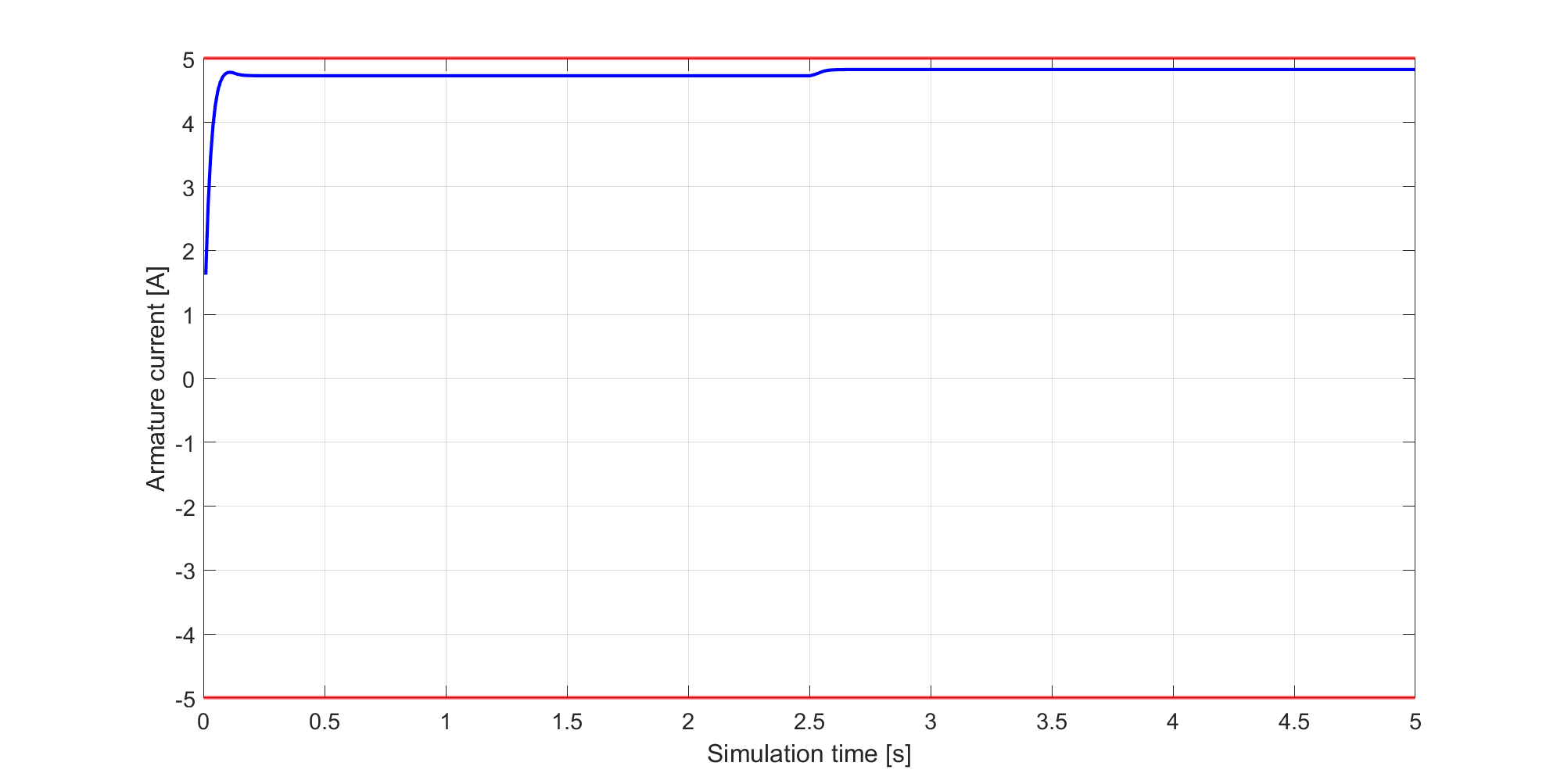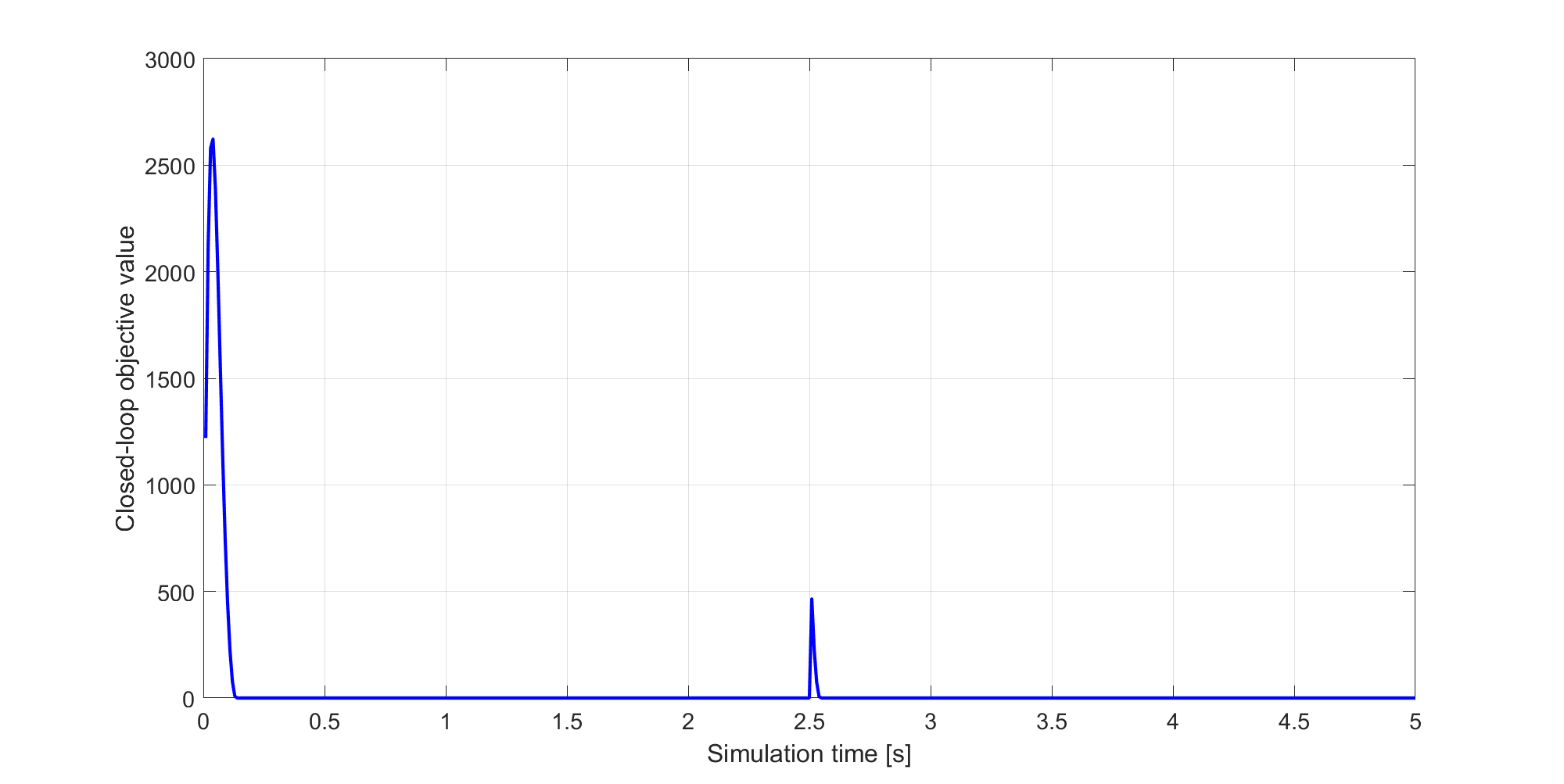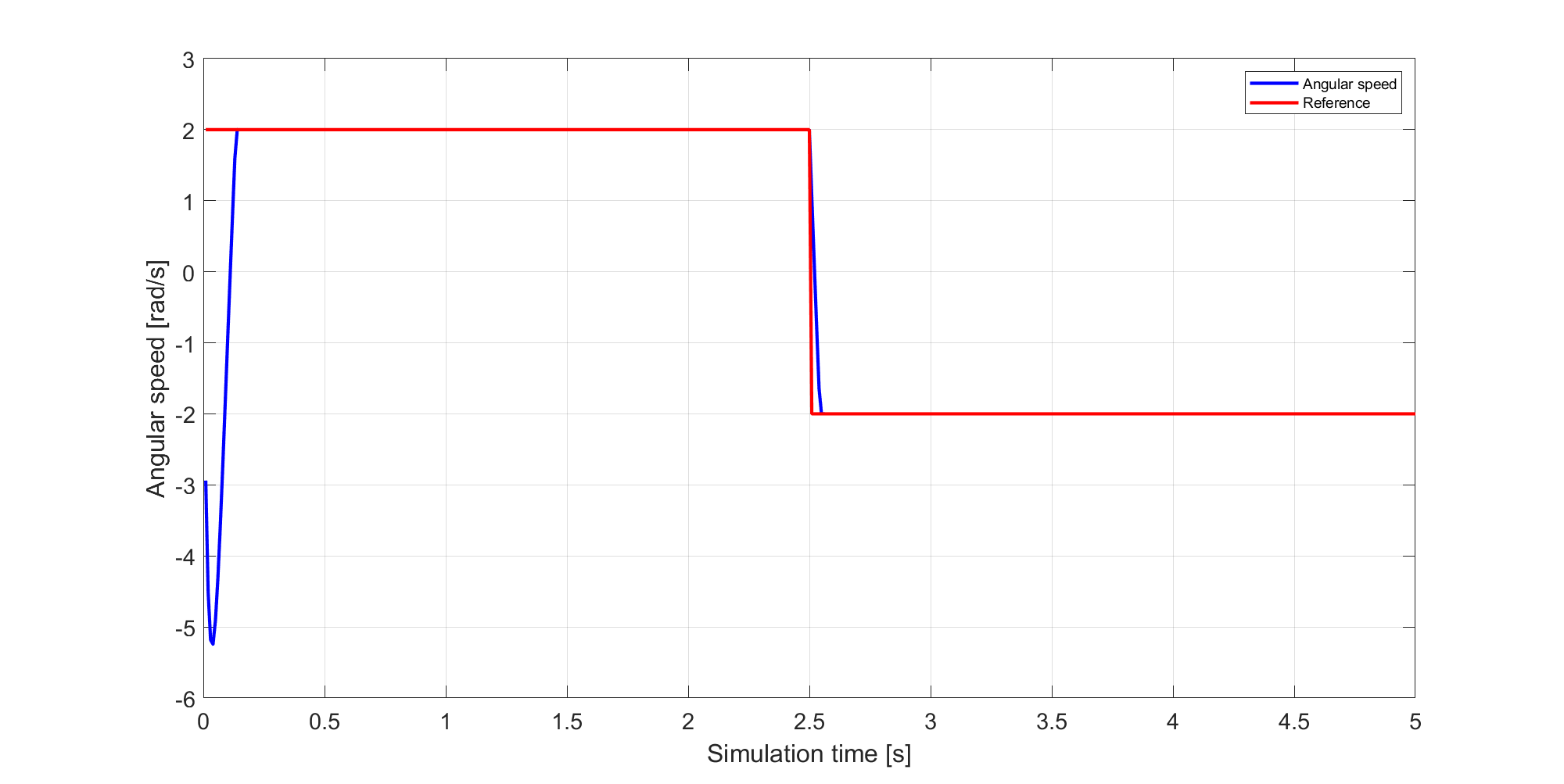# 11.17. Controlling a DC motor using a FORCESPRO SQP solver¶

In this example our aim is to control a DC-motor using a FORCESPRO SQP solver. The model for the DC motor which we consider is borrowed from [BerUnb], to which we refer for further details. The dynamics of our model is described by the following set of ordinary differential equations:

$\begin{split}\dot{x}_1(t) &= -\frac{R_a}{L_a}x_1(t) - \frac{k_m}{L_a}u(t)x_2(t) + \frac{u_a}{L_a} \\ \dot{x}_2(t) &= -\frac{B}{J}x_2(t) + \frac{k_m}{J}u(t)x_1(t) - \frac{\tau_l}{J}.\end{split}$

The states $$x_1$$ and $$x_2$$ model the armature current and motor angular speed of out DC motor respectively and the control $$u$$ models the input field current. The following values are chosen for our model constants

\begin{alignat*}{3} R_a &= 12.548 \Omega && \quad \text{(armature resistance)} \\ L_a &= 0.307 \text{H} && \quad \text{(armature inductance)} \\ k_m &= 0.23576 \text{Nm}/\text{A}^2 && \quad \text{(motor constant)} \\ u_a &= 60 \text{V} && \quad \text{(armature voltage)} \\ B &= 0.00783 \text{Nmsec} && \quad \text{(total viscous damping)} \\ \tau_L &= 1.47 \text{Nmsec} && \quad \text{(Load torque)} \\ J &= 0.00385 \text{Nmsec}^2 && \quad \text{(total moment of inertia)} \end{alignat*}

The control objective is to track a piecewise constant angular speed. To test the robustness of out resulting controller we switch reference half way through our simulation. In the first half of the simulation we will track a constant angular speed $$x_2^{ref1} = 2$$ and then switch to tracking a constant angular speed $$x_2^{ref2} = -2$$. We collect the 2-dimensional state vector $$x = (x_1, x_2)^T$$ and the 1-dimensional control $$u$$ in the vector

$\begin{split}z = \begin{pmatrix} u \\ x_1 \\ x_2 \end{pmatrix}\end{split}$

You can download the following MATLAB code of this example to try it out for yourself by clicking here.

## 11.17.1. Defining the MPC problem¶

### 11.17.1.1. The tracking objective function¶

Since we want to track a reference value it is natural to consider a least squared cost $$f(z,p) = \frac{|\!| r(z,p) |\!|^2}{2}$$ for

$r(z,p) = z_3 - p$

Recall that $$z_3=x_2$$ models the motor angular speed which is made to track a piecewise constant reference. The parameter $$p$$ will be equal to $$x_2^{ref1}$$ during the first half of the simulation and equal to $$x_2^{ref2}$$ during the second.

The following code snippet reads in the least squared objective

model.LSobjective = @(z,p) sqrt(100) * (z(3) - p);
model.LSobjectiveN = @(z,p) sqrt(100) * (z(3) - p);


### 11.17.1.2. The dynamics¶

The following code snippet reads in the dynamics (11.1) of our model:

%% model parameters
% Armature inductance (H)
La = 0.307;
% Armature resistance (Ohms)
Ra = 12.548;
% Motor constant (Nm/A^2)
km = 0.23576;
% Total moment of inertia (Nm.sec^2)
J = 0.00385;
% Total viscous damping (Nm.sec)
B = 0.00783;
tauL = 1.47;
% Armature voltage (V)
ua = 60;

model.E = [zeros(2,1), eye(2)];
model.continuous_dynamics = @(x,u) [(-1/La)*(Ra*x(1) + x(2)*u(1) - ua);...
(-1/J)*(B*x(2) - km*x(1)*u(1) + tauL)];


### 11.17.1.3. Input and state constraints¶

The following constraints are to be met by out control and state vectors:

$\begin{split}1 \text{A} \leq \ &u \leq 1.6 \text{A}\\ -5 \text{A} \leq \ &x_1 \leq 5 \text{A}\\ -10 \tfrac{\text{rad}}{\text{sec}} \leq \ &x_2 \leq 10 \tfrac{\text{rad}}{\text{sec}}\end{split}$

This can be read into the FORCESPRO model as follows

model.lb = [1, -5, -10];
model.ub = [1.6, 5, 10];


### 11.17.1.4. Generating the FORCESPRO SQP solver¶

To generate a suitable SQP solver for out MPC problem one need a model struct as well as a codeoptions struct. Our model struct has been populated above and we now specify the codeoptions we want and generating the solver by calling FORCES_NLP. The following code-snippet shows how this can be done:

%% set codeoptions
codeoptions = getOptions('FORCESPROSolver');
codeoptions.solvemethod = 'SQP_NLP'; % generate SQP solver
codeoptions.nlp.integrator.type = 'ERK4';
codeoptions.nlp.integrator.Ts = 0.01;
codeoptions.nlp.integrator.nodes = 1;
codeoptions.nlp.hessian_approximation = 'gauss-newton';
codeoptions.server = 'https://forces.embotech.com';

%% generate FORCESPRO solver
FORCES_NLP(model, codeoptions);


### 11.17.1.5. Calling the solver¶

Once the solver has been generated it needs a struct containing an initial guess, initial condition of the ODE, the run-time parameters and the reinitialize field as explained in Sequential quadratic programming algorithm. The following code-snippet shows how this can be done:

% populate run time parameters struct
params.all_parameters = repmat(2, model.N, 1);
params.xinit = zeros(model.neq, 1); % initial condition to ODE
params.x0 = repmat([1.2;zeros(2,1)], model.N, 1); % initial guess
params.reinitialize = 0;

% Solve optimization problem
[output, exitflag, info] = FORCESPROSolver(params);


The FORCESPROSolver is expected to return an exitflag equal to $$1$$, which corresponds to a successful solve. However, note that the QP could become infeasible in some cases. In this case, one should expect an exitflag equal to $$−8$$.

### 11.17.1.6. Results¶

The control objective is to track an angular speed of 2 and -2 respectively. As can be seen in Figure 11.57 the controller completes this task. The control ($$u$$) is plotted in Figure 11.53, the first state ($$x_1$$) is plotted in Figure 11.54 and second state ($$x_2$$) in Figure 11.55. As a measure of control quality, the closed loop objective value is plotted in Figure 11.56.Figure 11.53 The control ($$u$$, in blue) as a function of simulation time (s). The control obeys its constraints (red) throughout the simulation.¶Figure 11.54 The first state ($$x_1$$, in blue) as a function of simulation time. It obeys its constraints (red) throughout the simulation.¶Figure 11.55 The second state ($$x_2$$, in blue) as a function of simulation time. It obeys its constraints (red) throughout the simulation.¶Figure 11.56 Closed-loop objective value as a function of time¶Figure 11.57 Angular speed (blue) and tracked reference (red) value as a function of time.¶
[BerUnb]

S. Daniel-Berhe; H. Unbehauen: Experimental physical parameter estimation of a thyristor driven DC-motor using the HMF-method. Control Engineering Practice, 6:615–626, 1998